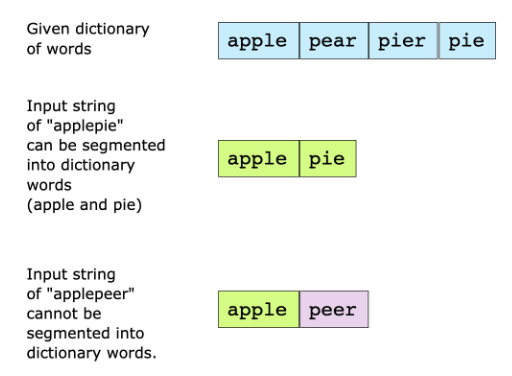# String Segmentation

## Problem Statement

You are given a dictionary of words and a large input string. You have to find out whether the input string can be completely segmented into the words of a given dictionary. The following two examples elaborate on the problem further.• Recursion
• Memoization

## Try it yourself

bool can_segment_string(string s, unordered_set<string> &dictionary) {  //TODO: Write - Your - Code  return false;}

## Solution

bool can_segment_string(string s, unordered_set <string> & dictonary) {  for (int i = 1; i <= s.length(); ++i) {    string first = s.substr(0, i);    if (dictonary.find(first) != dictonary.end()) {      string second = s.substr(i);      if (second.empty() || dictonary.find(second) != dictonary.end() || can_segment_string(second, dictonary)) {        return true;      }    }  }  return false;}int main() {  string s = "hellonow";  unordered_set <string> dictonary = { "hello", "hell", "on", "now" };  if (can_segment_string(s, dictonary))    cout << "String Can be Segmented" << endl;  else    cout << endl << "String Can NOT be Segmented" << endl;  return 0;}

## Solution Explanation

### Runtime Complexity

The runtime complexity of this solution is exponential, $O(2^{n})$, if we use only recursion.

With memoization, the runtime complexity of this solution can be improved to be polynomial, $O(n^{2})$

### Memory Complexity

The memory complexity of this solution is polynomial, $O(n^{2})$

### Solution Breakdown

You can solve this problem by segmenting the large string at each possible position to see if the string can be completely segmented to words in the dictionary. If you write the algorithm in steps it will be as follows:

n = length of input string
for i = 0 to n - 1
first_word = substring (input string from index [0, i] )
second_word = substring (input string from index [i + 1, n - 1] )
if dictionary has first_word
if second_word is in dictionary OR second_word is of zero length, then return true
recursively call this method with second_word as input and return true if it can be segmented


The algorithm will compute two strings from scratch in each iteration of the loop. Worst case scenario, there would be a recursive call of the second_word each time. This shoots the time complexity up to $2^{n}$.

You can see that you may be computing the same substring multiple times, even if it doesn’t exist in the dictionary. This redundancy can be fixed by memoization, where you remember which substrings have already been solved.

To achieve memoization, you can store the second string in a new set each time. This will reduce both time and memory complexities.

Practice problems like this and many more by checking out our Grokking the Coding Interview: Patterns for Coding Questions course!

Learn in-demand tech skills in half the time